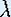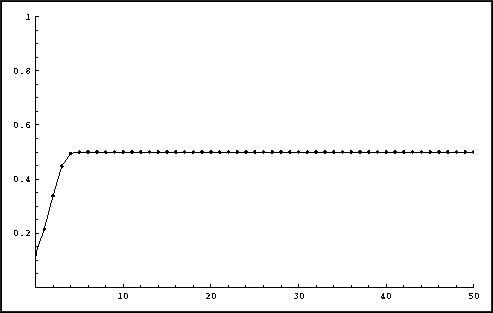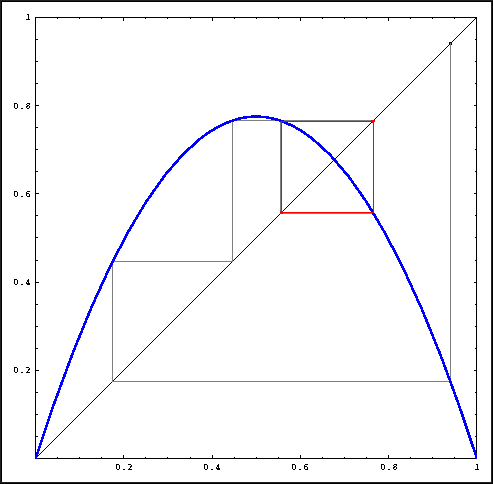Up: Iteration

# One-Dimensional Dynamical Systems

## Part 3: Iteration

#### Instructions for Mathematica: Iterations

First make sure that you have a personal copy of the package Chaos.m.

To start up Mathematica in a Unix window, type 'math', or 'mathematica' if your prefer the notebook version. On a PC, use the start button and find Mathematica in the Program directory. The Package written for this course is called 'Chaos.m'. Inside Mathematica, load this Package by typing

<< Chaos.m

When you load the Package, the file 'Logistic.m' is loaded automatically, so that the current function is set to the Logistic map

f(x) =x (1 - x).Function Iteration with= 2.

#### Instructions to Iterate

• Upon loading the Package, there are some messages printed. The last line says

lambda = 2, x0 = 0.94, its = 100

These are the variables and parameters that you can change. If you type

lambda = 3.1;

the parameteris set to 3.1. Similarly x0 is the initial value for which the orbit is computed, and its is the length of the computed orbit, that is, the number of iterates. (Note that x0 should be chosen in the closed interval [0,1].)

• Next, type

PlotIterates

A figure will appear with the plot of the iterates versus time.

• You may wish to Look at only part of this picture, especially when the number of iterates is large. Notice that the figure has a number, for example

Out

If you type

Show[Out, PlotRange -> {{50, 100}, {0, 1}}]

A new figure appears with only the 50-th to the 100-th iterates. You can use the shortcuts % and %% for the numbers of the last and next to last output lines.

• Experiment with different values forand x0.

#### Instructions for Mathematica: Graphical Iteration

First make sure that you have a personal copy of the package Chaos.m.

To start up Mathematica in a Unix window, type 'math', or 'mathematica' if your prefer the notebook version. On a PC, use the start button and find Mathematica in the Program directory. The Package written for this course is called 'Chaos.m'. Inside Mathematica, load this Package by typing

<< Chaos.m

For the Logistic map, pick avalue between 0 and 4, and iterate as follows:Visualizing an orbit with graphical iteration for the Logistic map.

#### Instructions to Iterate

• You can change the variable x0 and parameters lambda and its by typing something like

x0 = 0.12345;

• Next, type

PlotGraphical

A figure will appear with the graph of the Logistic map for this specificvalue. The line y = x is drawn as well, and you see the graphical iteration of x0 with the last iterates drawn in red. The actual points of the eventual behavior are drawn as thick red dots on the diagonal.

• You may wish to zoom in to this picture. For this, type

Show[%, PlotRange -> {{0.6, 0.8}, {0.6, 0.8}}]

A new figure appears of only the box [0.6, 0.8] X [0.6, 0.8]. You can type something similar to zoom in more, but also to zoom out, even though the last picture does not show all points.

Up: IterationThe Geometry Center Home Page

Written by Hinke Osinga